PSAJ2E - Grade 8 Mathematics Practice Test 2000
What does y equal in the equation below?

6 - 2y = -8

Modified from EngageNY ©Great Minds Disclaimer

The formula for the surface area (SA) of a cube is SA = 6e2,
where e is the length of an edge of the cube.
An edge of a red cube is twice as long as an edge of a blue cube.
How many times greater is the surface area of the red cube than that of the blue cube?

Modified from EngageNY ©Great Minds Disclaimer

In the figure, the perimeter of the equilateral triangle is 24 inches. What is the area of the square?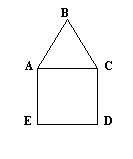Modified from EngageNY ©Great Minds Disclaimer

The Massachusetts Department of Employment and Training predicted that there will be 21% more jobs for computer programmers in Massachusetts in 2005 than there were in 1994. There were 15,970 programming jobs in 1994. Based on the prediction, approximately how many new jobs will be available by 2005?

Modified from EngageNY ©Great Minds Disclaimer

Type your answer below as a number (example: 5, 3.1, 4 1/2, or 3/2):
What number comes next in this sequence?
5, 6, 9, 14, 21, ____
The above figure is to be rotated 90 degrees clockwise. What would the figure look like after the rotation?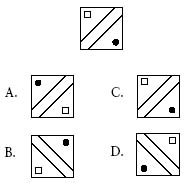A bag contains 3 red, 2 green, and 4 blue balls. John is going to draw out a ball without looking in the bag. What is the probability that he will draw either a green or a blue ball?
A chemical solution is 0.5% iodine. How many milliliters of iodine are in 1,000 mL of solution?
This is a regular hexagon.
How many degrees are in angle EFG?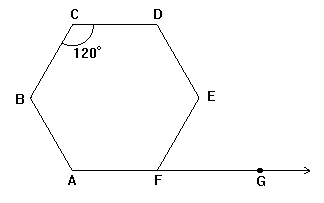Write an expression that tells how much money a shopper has left if x represents the amount the shopper started with and s represents the amount spent.

Modified from EngageNY ©Great Minds Disclaimer

How many cylinders must be placed on the empty side of the second scale to make that scale balance?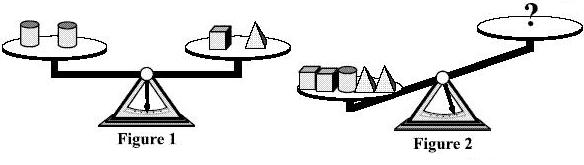Modified from EngageNY ©Great Minds Disclaimer

Type your answer below as a number (example: 5, 3.1, 4 1/2, or 3/2):
Compute:

43.68 x 2.5 = ?

Modified from EngageNY ©Great Minds Disclaimer

Myra drew a square. She drew another square inside the first square as shown in figure A.
Then she drew a square inside her second square, a square inside her third square, and so on. After she had drawn five squares, her figure looked like figure B.
Write an expression that tells the number of right angles in Myra's figure after she drew exactly n squares.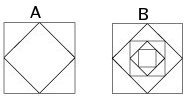Modified from EngageNY ©Great Minds Disclaimer

The formula for the area, A, of a square is:

A = s2, where s is the length of the side of the square.

Which graph shows the relationship between the length of one side of a square and its area?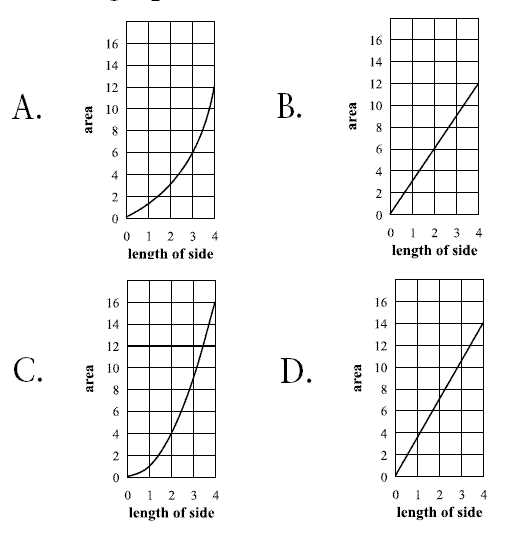Select one:

Find the rule for the table below.

 Input (x) Output (y) 3 -5 6 -11 2 -3 8 -15

What does y equal in terms of x?

Your answer should be the expression that goes in the space for y = ________________ .

Do not type in y = .

Modified from EngageNY ©Great Minds Disclaimer